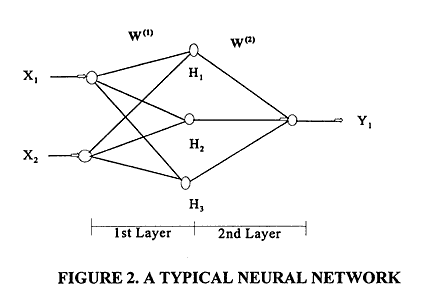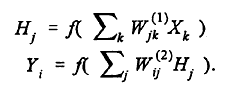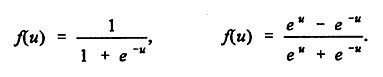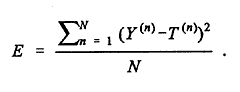U.S. Department of Transportation
1200 New Jersey Avenue, SE
Washington, DC 20590
202-366-4000

Federal Highway Administration Research and Technology
Coordinating, Developing, and Delivering Highway Transportation InnovationsThis report is an archived publication and may contain dated technical, contact, and link information
 Federal Highway Administration > Publications > Research Publications > 98133 > Ch02_05.Cfm > Accident Models for Two-Lane Rural Roads: Segment and Intersections
Publication Number: FHWA-RD-98-133
Date: October 1998

# Accident Models for Two-Lane Rural Roads: Segment and Intersections

## 2. Literature Review

Artificial Neural Networks

Artificial neural network applications have recently received considerable attention. The methodology of modeling, or estimation, is somewhat comparable to statistical modeling (Smith, 1993). Neural networks should not, however, be heralded as a substitute for statistical modeling, but rather as a complementary effort (without the restrictive assumption of a particular statistical model) or an alternative approach to fitting non-linear data.

A typical neural network (shown in Figure 2) is composed of input units X1, X2, ... corresponding to independent variables (in our case, highway or intersection variables), a hidden layer known as the first layer, and an output layer (second layer) whose output units Y1, ... correspond to dependent variables (expected number of accidents per time period).In between are hidden units H1, H2, ... corresponding to intermediate variables. These interact by means of weight matrices W(1) and W(2) with adjustable weights. The values of the hidden units are obtained from the formulas:One multiplies the first weight matrix by the input vector X = (X1, X2, ...), and then applies an activation function f to each component of the result. Likewise the values of the output units are obtained by applying the second weight matrix to the vector H = (H1, H2, ...) of hidden unit values, and then applying the activation function f to each component of the result. In this way one obtains an output vector Y= (Y1, Y2, ...).

The activation function f is typically of sigmoid form and may be a logistic function, hyperbolic tangent, etc.:Usually the activation function is taken to be the same for all components but it need not be.

Values of W(1) and W(2) are assumed at the initial iteration. The accuracy of the estimated output is improved by an iterative learning process in which the outputs for various input vectors are compared with targets (observed frequency of accidents) and an average error term E is computed:Here

N = Number of highway sites or observations

Y(n) = Estimated number of accidents at site n for n = 1, 2, ..., N

T(n) = Observed number of accidents at site n for n = 1, 2, ..., N.

After one pass through all observations (the training set), a gradient descent method may be used to calculate improved values of the weights W(1) and W(2), values that make E smaller. After reevaluation of the weights with the gradient descent method, successive passes can be made and the weights further adjusted until the error is reduced to a satisfactory level. The computation thus has two modes, the mapping mode, in which outputs are computed, and the learning mode, in which weights are adjusted to minimize E. Although the method may not necessarily converge to a global minimum, it generally gets quite close to one if an adequate number of hidden units are employed.

The most delicate part of neural network modeling is generalization, the development of a model that is reliable in predicting future accidents. Overfitting (i.e., getting weights for which E is so small on the training set that even random variation is accounted for) can be minimized by having two validation samples in addition to the training sample. According to Smith (1993), the data set should be divided into three subsets: 40% for training, 30% to prevent overfitting, and 30% for testing. Training on the training set should stop at the epoch when the error E computed on the second set begins to rise (the second set is not used for training but merely to decide when to stop training). Then the third set is used to see how well the model performs. The cross-validation helps to optimize the fit in three ways: by limiting/optimizing the number of hidden units, by limiting/optimizing the number of iterations, and by inhibiting network use of large weights.

The major advantages and disadvantages of neural networks in modeling applications are as follows:

· There is no need to assume an underlying data distribution such as usually is done in statistical modeling.

· Neural networks are applicable to multivariate non-linear problems.

· The transformations of the variables are automated in the computational process.

· Minimizing overfitting requires a great deal of computational effort.

· The individual relations between the input variables and the output variables are not developed by engineering judgment so that the model tends to be a black box or input/output table without analytical basis.

· The sample size has to be large.

The disadvantages appear to outweigh the advantages, particularly in view of the black box effect.